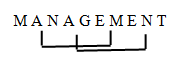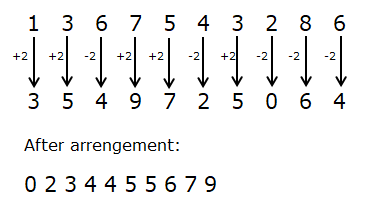# SBI Clerk Prelims Reasoning Ability Questions 2021 (Day-47)

Dear Aspirants, Our IBPS Guide team is providing new series of Reasoning Questions for SBI Clerk Prelims 2021 so the aspirants can practice it on a daily basis. These questions are framed by our skilled experts after understanding your needs thoroughly. Aspirants can practice these new series questions daily to familiarize with the exact exam pattern and make your preparation effective.

Start Quiz

Miscellaneous

1) If the position of the letters in the word “CORPORATION” are rearranged in such a way that the position of N remains same position, then the first and the tenth letter are interchanged, similarly the position of the second and ninth letters are interchanged and so on, from the left end then which of the following will be the sixth from the left end after the rearrangement?

A.R

B.C

C.O

D.P

E.A

2) How many such pairs of letters are there in the word “MANAGEMENT” each of which has as many letters between them in the word as in the English alphabets (Both backward and forward direction)?

A.1

B.3

C.4

D.2

E.More than four

3) If in a number ‘1367543286’ two is added in each odd digit and two is subtracted from each even digit after that arrange it in ascending order from left to right. After the operation which of the following digit will be fifth from right end?

A.6

B.5

C.4

D.3

E.None of these

Alphanumeric series

Direction (4-7): The questions are based on the following arrangement. Study the arrangement carefully to answer these questions.

P  6  E  1  @  L  A  9  \$  G  3  S  2  U  #  M  8  O  4  &  E  9  *  H  5  R

4) How many perfect squares are present in the above series which are immediately followed by a symbol and immediately preceded by a vowel?

A.1

B.2

C.3

D.4

E.More than four

5) If all the numbers will remove in above series then which element will be fourth to the left of sixth from right end?

A.S

B.U

C.G

D.\$

E.None of these

6) How many letters are present in the above series which are immediately followed by a number and immediately preceded by a symbol?

A.None

B.1

C.2

D.3

E.More than three

7) How many numbers are present in the above series which are immediately followed by a vowel and immediately preceded by a consonant?

A.1

B.2

C.3

D.4

E.None of these

Inequality

Direction (8-10): In each of the following questions, relationship between different elements is shown in the statements followed by two conclusions. Find the conclusion which is definitely true.

a)If only conclusion I follows.

b)If only conclusion II follows.

c)If either conclusion I or II follows.

d)If neither conclusion I nor II follows.

e)If both conclusions I and II follow.

8) Statements:

M < L > V ≤ C; K ≥ N = T ≤ P; G ≥ L < N ≥ H

Conclusion:

I)P > V

II)G ≥ M

9) Statements:

P ≤ Z < E < T; R ≤ S = A < U; P ≥ U ≤ D

Conclusion:

I)T > A

II)R < D

10) Statements:

L < N ≥ C = K; G ≥ M ≤ T = K

Conclusion:

I)N > M

II) M = N

CORPORATION

OITAROPROCN[email protected], A9\$, O4&, E9*

P  E  @  L  A  \$  G   U  #  M  O  &  E  *  H  R

\$G3, #M8, *H5, &E9

P6E, S2U, M8O

I) P > V (True) (P ≥ T = N > L > V)

II) G ≥ M (False) (G ≥ L > M)

I) T > A (True) (T > E > Z ≥ P ≥U > A

II) R < D (True) (D ≥ U > A = S ≥ R)